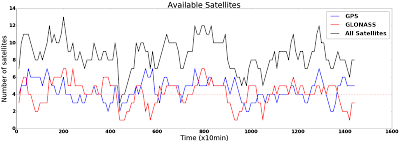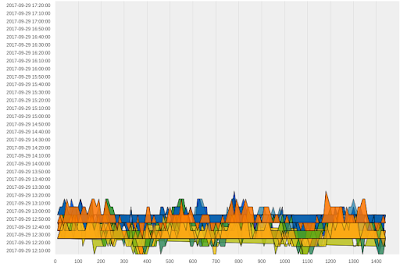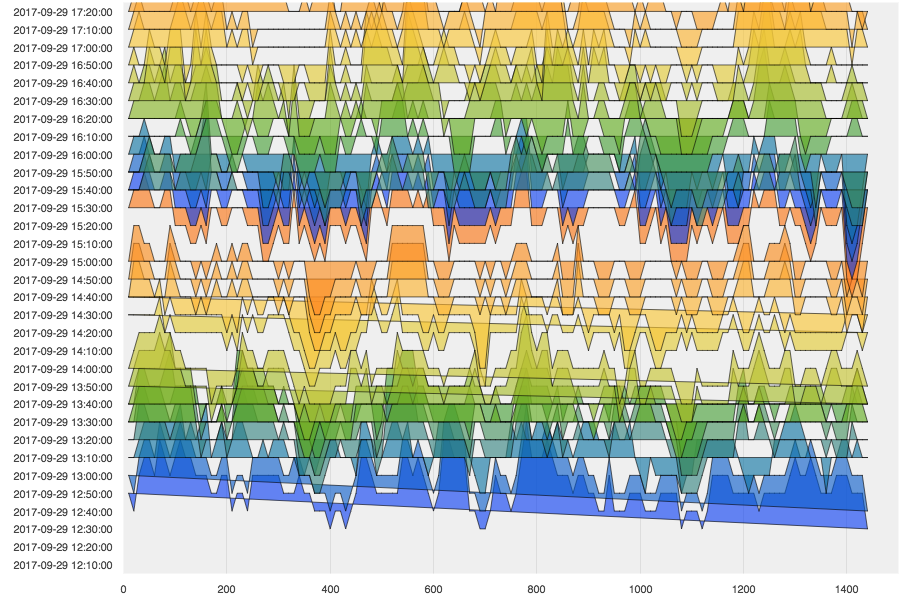# Joyplot in Bokeh

Hi every one, I’am trying to show this:This image represent how much satellites are in a point (longitude-latitude) through the day. Axis X is a whole day in minutes and Axis Y is quantity of satellites in that moment.

So I want to do this chart (just for GPS, I mean the blue one) in differents days. I was thinking represent this with a Joyplot. Using the code that attach I get the next figure.

Something I am wrong… It supposed each category is a day (same day, but different time) but seems is all are in the same category.my code is:

from numpy import linspace
import bokeh
from scipy.stats.kde import gaussian_kde
from bokeh.io import output_file, show
from bokeh.models import ColumnDataSource, FixedTicker, PrintfTickFormatter
from bokeh.plotting import figure
#from bokeh.sampledata.perceptions import probly
bokeh.BOKEH_RESOURCES=‘inline’
import colorcet as cc

output_file(“joyplot.html”)
cats = g #ListOfTime # list(reversed(probly.keys())) #list([‘Pos_1’,‘Pos_2’]) #
print len(cats),’ lengh of times’
palette = [cc.rainbow[i*15] for i in range(16)]
palette += palette
print len(palette),‘lengh palette’
x = X # linspace(-20,110, 500) #Test.X #
print len(x),’ lengh X’
source = ColumnDataSource(data=dict(x=x))
p = figure(y_range=cats, plot_width=900, x_range=(0, 1500), toolbar_location=None)
for i, cat in enumerate(reversed(cats)):
y = EveryTest[i].GPS #joy(cat, pdf(x))
#print i,cat
p.patch(‘x’, cat, color=palette[i], alpha=0.6, line_color=“black”, source=source)
#break
#print source

p.outline_line_color = None
p.background_fill_color = “#efefef
p.ygrid.grid_line_color = None
p.xgrid.grid_line_color = “#dddddd
#p.xgrid.ticker = p.xaxis.ticker
p.axis.minor_tick_line_color = None
p.axis.major_tick_line_color = None
p.axis.axis_line_color = None
show§

``

Hi,

down votefavorite

I fixed one part of the code that was wrong, With that line of code, I add the category for every information (Axis X).

``````y = joy(cat, EveryTest[i].GPS)
``````

After to add that line of code, the graph improved, but still its failing something. the graph starts with the 4th category (I mean 12:40:00), and it must start in the first (12:10:00), What I am doing wrong?···

El martes, 12 de diciembre de 2017, 15:26:32 (UTC-5), Michael Sneider escribió:

Hi every one, I’am trying to show this:This image represent how much satellites are in a point (longitude-latitude) through the day. Axis X is a whole day in minutes and Axis Y is quantity of satellites in that moment.

So I want to do this chart (just for GPS, I mean the blue one) in differents days. I was thinking represent this with a Joyplot. Using the code that attach I get the next figure.

Something I am wrong… It supposed each category is a day (same day, but different time) but seems is all are in the same category.my code is:

from numpy import linspace
import bokeh
from scipy.stats.kde import gaussian_kde
from bokeh.io import output_file, show
from bokeh.models import ColumnDataSource, FixedTicker, PrintfTickFormatter
from bokeh.plotting import figure
#from bokeh.sampledata.perceptions import probly
bokeh.BOKEH_RESOURCES=‘inline’
import colorcet as cc

output_file(“joyplot.html”)
cats = g #ListOfTime # list(reversed(probly.keys())) #list([‘Pos_1’,‘Pos_2’]) #
print len(cats),’ lengh of times’
palette = [cc.rainbow[i*15] for i in range(16)]
palette += palette
print len(palette),‘lengh palette’
x = X # linspace(-20,110, 500) #Test.X #
print len(x),’ lengh X’
source = ColumnDataSource(data=dict(x=x))
p = figure(y_range=cats, plot_width=900, x_range=(0, 1500), toolbar_location=None)
for i, cat in enumerate(reversed(cats)):
y = EveryTest[i].GPS #joy(cat, pdf(x))
#print i,cat
p.patch(‘x’, cat, color=palette[i], alpha=0.6, line_color=“black”, source=source)
#break
#print source

p.outline_line_color = None
p.background_fill_color = “#efefef
p.ygrid.grid_line_color = None
p.xgrid.grid_line_color = “#dddddd
#p.xgrid.ticker = p.xaxis.ticker
p.axis.minor_tick_line_color = None
p.axis.major_tick_line_color = None
p.axis.axis_line_color = None
show§

``

Can your series have both positive and negative values? The original example code is simple and assumes probability distributions, which are always positive and are also zero at both ends. The patches connects the first and last points of the series, so if the fist and last points have different y values, you'll get diagonal lines such as you see.

Bryan

···

On Dec 13, 2017, at 13:00, Michael Sneider <[email protected]> wrote:

Hi,

down vote
favorite

I fixed one part of the code that was wrong, With that line of code, I add the category for every information (Axis X).

y = joy(cat, EveryTest[i].GPS)
After to add that line of code, the graph improved, but still its failing something. the graph starts with the 4th category (I mean 12:40:00), and it must start in the first (12:10:00), What I am doing wrong?

El martes, 12 de diciembre de 2017, 15:26:32 (UTC-5), Michael Sneider escribió:
Hi every one, I'am trying to show this:

This image represent how much satellites are in a point (longitude-latitude) through the day. Axis X is a whole day in minutes and Axis Y is quantity of satellites in that moment.

So I want to do this chart (just for GPS, I mean the blue one) in differents days. I was thinking represent this with a Joyplot. Using the code that attach I get the next figure.

Something I am wrong... It supposed each category is a day (same day, but different time) but seems is all are in the same category.

my code is:

from numpy import linspace
import bokeh
from scipy.stats.kde import gaussian_kde
from bokeh.io import output_file, show
from bokeh.models import ColumnDataSource, FixedTicker, PrintfTickFormatter
from bokeh.plotting import figure
#from bokeh.sampledata.perceptions import probly
bokeh.BOKEH_RESOURCES='inline'
import colorcet as cc

output_file("joyplot.html")
cats = g #ListOfTime # list(reversed(probly.keys())) #list(['Pos_1','Pos_2']) #
print len(cats),' lengh of times'
palette = [cc.rainbow[i*15] for i in range(16)]
palette += palette
print len(palette),'lengh palette'
x = X # linspace(-20,110, 500) #Test.X #
print len(x),' lengh X'
source = ColumnDataSource(data=dict(x=x))
p = figure(y_range=cats, plot_width=900, x_range=(0, 1500), toolbar_location=None)
for i, cat in enumerate(reversed(cats)):
y = EveryTest[i].GPS #joy(cat, pdf(x))
#print i,cat
p.patch('x', cat, color=palette[i], alpha=0.6, line_color="black", source=source)
#break
#print source

p.outline_line_color = None
p.background_fill_color = "#efefef"
p.ygrid.grid_line_color = None
p.xgrid.grid_line_color = "#dddddd"
#p.xgrid.ticker = p.xaxis.ticker
p.axis.minor_tick_line_color = None
p.axis.major_tick_line_color = None
p.axis.axis_line_color = None
show(p)

--
You received this message because you are subscribed to the Google Groups "Bokeh Discussion - Public" group.
To unsubscribe from this group and stop receiving emails from it, send an email to [email protected].
To post to this group, send email to [email protected].
To view this discussion on the web visit https://groups.google.com/a/continuum.io/d/msgid/bokeh/b9f90a5b-01e3-448d-ba9d-48ea807e9da7%40continuum.io.

The original image you said you were trying to show just had lines and not filled areas. If that suits your case then I would suggest using p.line instead of p.patch. The line version will not try to "connect up" the first and last points.

Thanks,

Bryan

···

On Dec 13, 2017, at 13:00, Michael Sneider <[email protected]> wrote:

Hi,

down vote
favorite

I fixed one part of the code that was wrong, With that line of code, I add the category for every information (Axis X).

y = joy(cat, EveryTest[i].GPS)
After to add that line of code, the graph improved, but still its failing something. the graph starts with the 4th category (I mean 12:40:00), and it must start in the first (12:10:00), What I am doing wrong?

El martes, 12 de diciembre de 2017, 15:26:32 (UTC-5), Michael Sneider escribió:
Hi every one, I'am trying to show this:

This image represent how much satellites are in a point (longitude-latitude) through the day. Axis X is a whole day in minutes and Axis Y is quantity of satellites in that moment.

So I want to do this chart (just for GPS, I mean the blue one) in differents days. I was thinking represent this with a Joyplot. Using the code that attach I get the next figure.

Something I am wrong... It supposed each category is a day (same day, but different time) but seems is all are in the same category.

my code is:

from numpy import linspace
import bokeh
from scipy.stats.kde import gaussian_kde
from bokeh.io import output_file, show
from bokeh.models import ColumnDataSource, FixedTicker, PrintfTickFormatter
from bokeh.plotting import figure
#from bokeh.sampledata.perceptions import probly
bokeh.BOKEH_RESOURCES='inline'
import colorcet as cc

output_file("joyplot.html")
cats = g #ListOfTime # list(reversed(probly.keys())) #list(['Pos_1','Pos_2']) #
print len(cats),' lengh of times'
palette = [cc.rainbow[i*15] for i in range(16)]
palette += palette
print len(palette),'lengh palette'
x = X # linspace(-20,110, 500) #Test.X #
print len(x),' lengh X'
source = ColumnDataSource(data=dict(x=x))
p = figure(y_range=cats, plot_width=900, x_range=(0, 1500), toolbar_location=None)
for i, cat in enumerate(reversed(cats)):
y = EveryTest[i].GPS #joy(cat, pdf(x))
#print i,cat
p.patch('x', cat, color=palette[i], alpha=0.6, line_color="black", source=source)
#break
#print source

p.outline_line_color = None
p.background_fill_color = "#efefef"
p.ygrid.grid_line_color = None
p.xgrid.grid_line_color = "#dddddd"
#p.xgrid.ticker = p.xaxis.ticker
p.axis.minor_tick_line_color = None
p.axis.major_tick_line_color = None
p.axis.axis_line_color = None
show(p)

--
You received this message because you are subscribed to the Google Groups "Bokeh Discussion - Public" group.
To unsubscribe from this group and stop receiving emails from it, send an email to [email protected].
To post to this group, send email to [email protected].
To view this discussion on the web visit https://groups.google.com/a/continuum.io/d/msgid/bokeh/b9f90a5b-01e3-448d-ba9d-48ea807e9da7%40continuum.io.

This is the answer,I could not see it before. that is the reason, I do not have an area, just a line. thank you Bryan .

···

2017-12-13 14:11 GMT-05:00 Bryan Van de ven [email protected]:

The original image you said you were trying to show just had lines and not filled areas. If that suits your case then I would suggest using p.line instead of p.patch. The line version will not try to “connect up” the first and last points.

Thanks,

Bryan

On Dec 13, 2017, at 13:00, Michael Sneider [email protected] wrote:

Hi,

down vote

favorite

I fixed one part of the code that was wrong, With that line of code, I add the category for every information (Axis X).

y = joy(cat, EveryTest[i].GPS)

After to add that line of code, the graph improved, but still its failing something. the graph starts with the 4th category (I mean 12:40:00), and it must start in the first (12:10:00), What I am doing wrong?

El martes, 12 de diciembre de 2017, 15:26:32 (UTC-5), Michael Sneider escribió:

Hi every one, I’am trying to show this:

This image represent how much satellites are in a point (longitude-latitude) through the day. Axis X is a whole day in minutes and Axis Y is quantity of satellites in that moment.

So I want to do this chart (just for GPS, I mean the blue one) in differents days. I was thinking represent this with a Joyplot. Using the code that attach I get the next figure.

Something I am wrong… It supposed each category is a day (same day, but different time) but seems is all are in the same category.

my code is:

from numpy import linspace

import bokeh

from scipy.stats.kde import gaussian_kde

from bokeh.io import output_file, show

from bokeh.models import ColumnDataSource, FixedTicker, PrintfTickFormatter

from bokeh.plotting import figure

#from bokeh.sampledata.perceptions import probly

bokeh.BOKEH_RESOURCES=‘inline’

import colorcet as cc

output_file(“joyplot.html”)

cats = g #ListOfTime # list(reversed(probly.keys())) #list([‘Pos_1’,‘Pos_2’]) #

print len(cats),’ lengh of times’

palette = [cc.rainbow[i*15] for i in range(16)]

palette += palette

print len(palette),‘lengh palette’

x = X # linspace(-20,110, 500) #Test.X #

print len(x),’ lengh X’

source = ColumnDataSource(data=dict(x=x))

p = figure(y_range=cats, plot_width=900, x_range=(0, 1500), toolbar_location=None)

for i, cat in enumerate(reversed(cats)):

``````y = EveryTest[i].GPS #joy(cat, pdf(x))
``````
``````#print i,cat
``````
``````source.add(y,cat)
``````
``````p.patch('x', cat, color=palette[i], alpha=0.6, line_color="black", source=source)
``````
``````#break
``````

#print source

p.outline_line_color = None

p.background_fill_color = “#efefef

p.ygrid.grid_line_color = None

p.xgrid.grid_line_color = “#dddddd

#p.xgrid.ticker = p.xaxis.ticker

p.axis.minor_tick_line_color = None

p.axis.major_tick_line_color = None

p.axis.axis_line_color = None

show§

You received this message because you are subscribed to the Google Groups “Bokeh Discussion - Public” group.

To unsubscribe from this group and stop receiving emails from it, send an email to [email protected].

To post to this group, send email to [email protected].

To view this discussion on the web visit https://groups.google.com/a/continuum.io/d/msgid/bokeh/b9f90a5b-01e3-448d-ba9d-48ea807e9da7%40continuum.io.